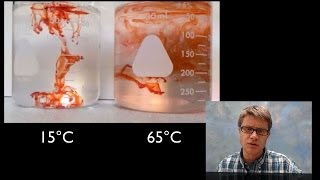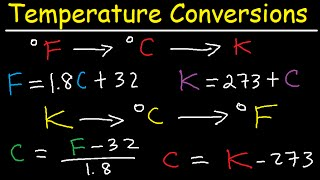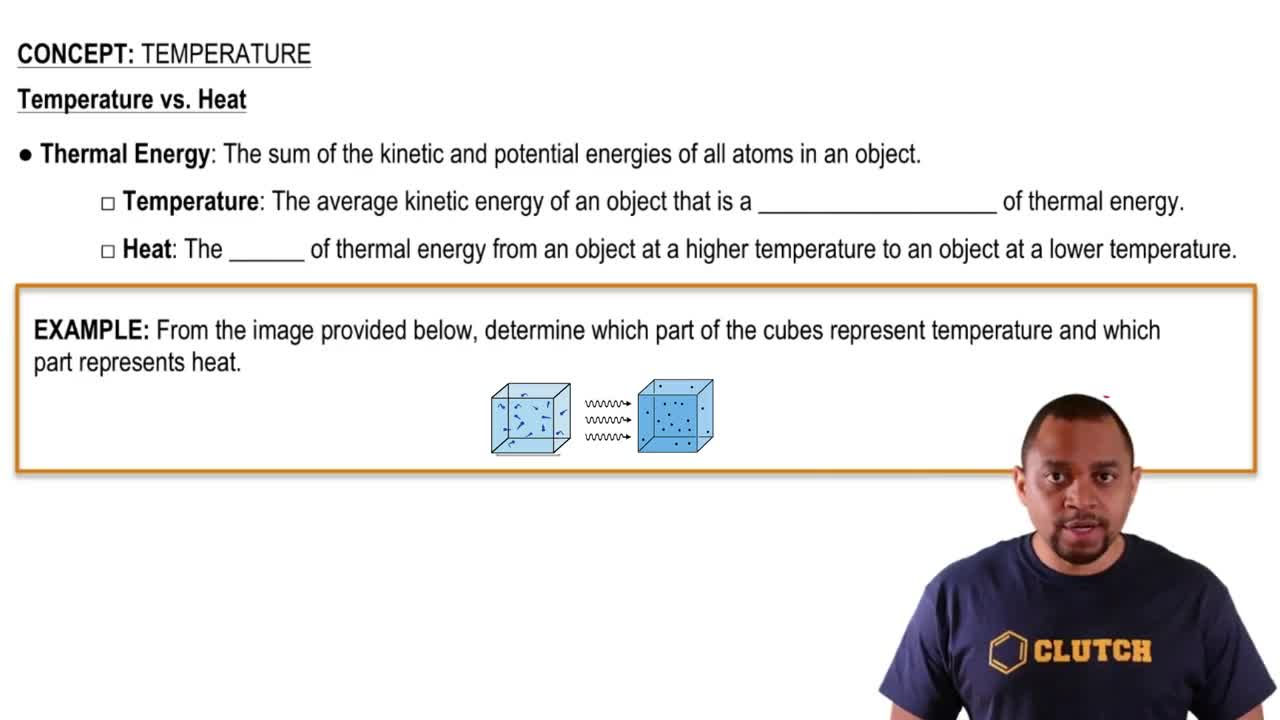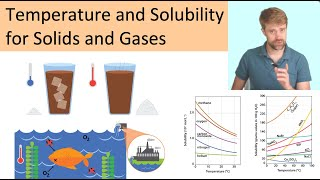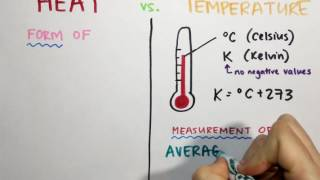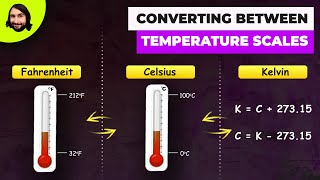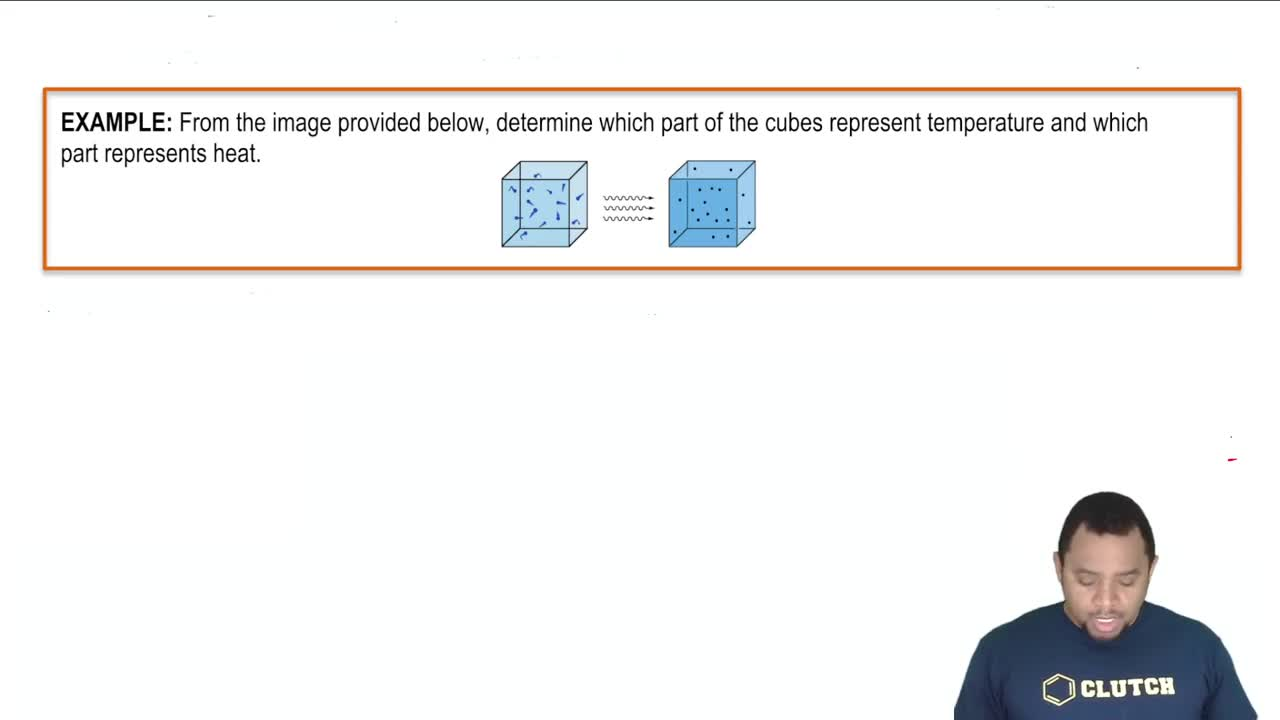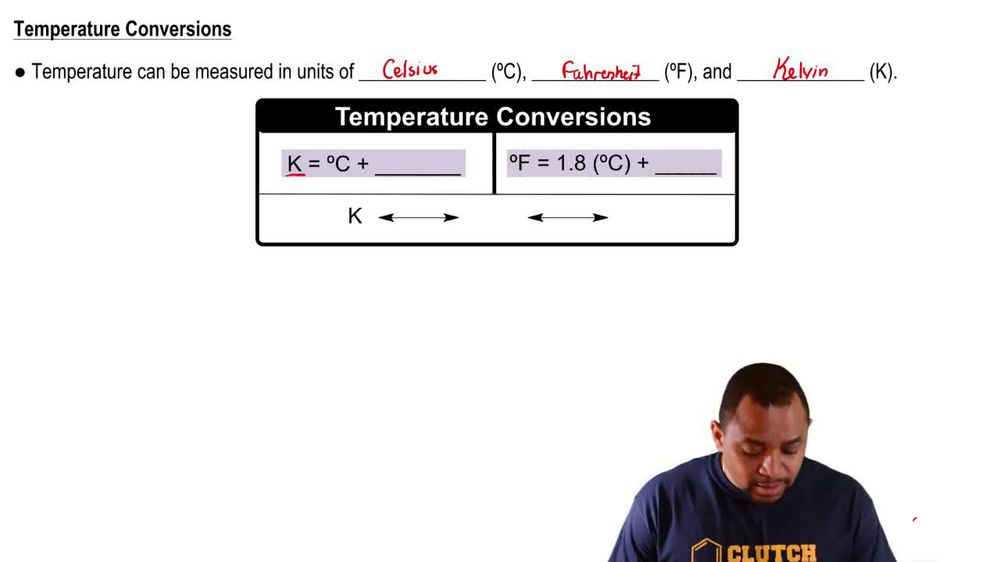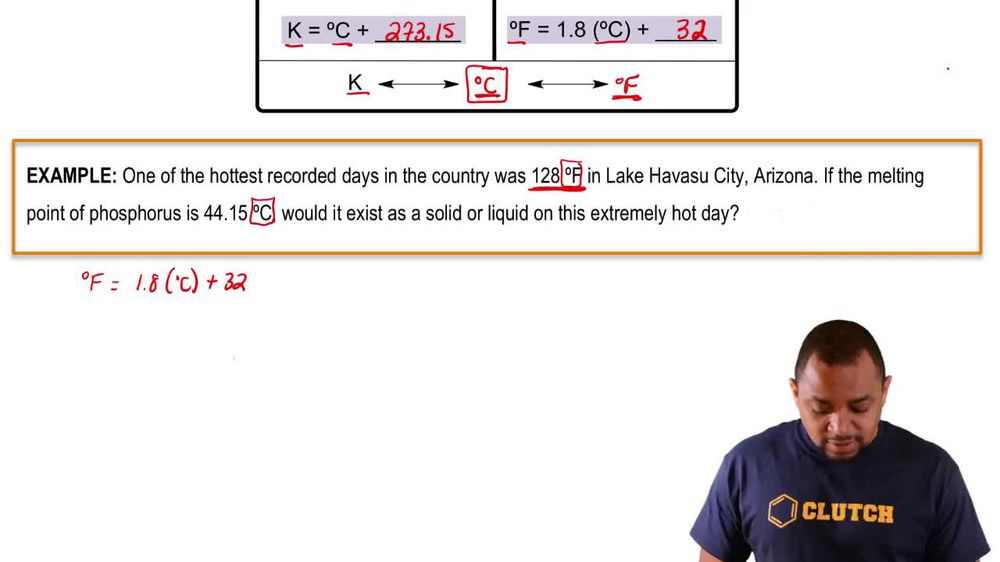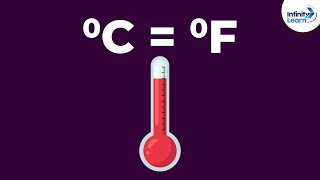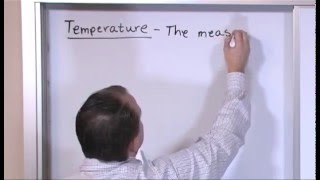Start typing, then use the up and down arrows to select an option from the list.
1. 1. Intro to General Chemistry2. Temperature
Problem

# Suppose you were dissatisfied with both Celsius and Fahrenheit units and wanted to design your own temperature scale based on ethyl alcohol (ethanol). On the Celsius scale, ethanol has a melting point of -117.3 °C and a boiling point of 78.5 °C, but on your new scale calibrated in units of degrees ethanol, °E, you define ethanol to melt at 0 °E and boil at 200 °E. (c) What are the melting and boiling points of water on the ethanol scale?

Relevant Solution1m
Play a video:
Hey everyone, let's practice our knowledge on boiling points and converting our temperatures for the first part, we're asked To convert our boiling point of 64.7°C into units of Fahrenheit. To answer this question, we need to recall the following equation. So we have our degrees Fahrenheit is equal to 1.8 Or you might also see it as 9/5 times are degrees Celsius And we're going to add 32. So plugging in our values, we get 1.8 Times 64.7°C plus 32. And that gets us to A temperature of .46°F. Now for part B, it's asking us whether methanol is a solid liquid or gas on a sunny F date. Thinking about this, we know that it won't be a gas Because we're way below our boiling point of 148°. Also, we know that we can't be a solid Because we're way above our freezing point of negative 143°. So that must mean Methanol is a liquid at 75°F. So this is going to be our final answers and I hope that made sense. But let us know if you have any questions